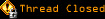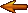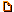# Topic awaiting preservation: some more maths... (Page 1 of 1)Angel's Demon
Neurotic (0) Inmateposted 07-09-2003 08:04

you gus seem to be really on top of things in the math department... so let me ask you a probalem that i am stuck in.

the original problem is:

x^5-10x+5=x^.5 2x= 4^x

i just put 2 in for the hell of it, too see if it worked out, and this is what i got.

(2)^5 - 10(2) + 5 = (2)^.5 - 2(2)^2 = 4^(2)

so then i go simplifing it...

(36 - 20) + 5 = 2^.5 -8 = 16

16 + 5 = 2^ .5 - 8 = 16

21 = 2^.5 -8 = 16

5 = 2^.5 -8

and then i am left with:

13 = 2^.5 <<<<<<< which doesnt make sense

i checked in on the calculator and i got 4, but somehow it doesn't make sense.

i was wondering if any of you guys could help me out.

>>>>>>>>>><<<<<<<<<<<<<>>>>>>>>>><<<<<<<<<<<< <<>>>>>>>>>

and just in case any of you are wondering who i am, and how i got here... i'm "petskull's" little sis.

Yannah
Paranoid (IV) Inmate

From: In your Hard Drive; C:
Insane since: Dec 2002posted 07-09-2003 08:15

oh Hi there Petskull's little sis.

I don't want to solve that problem right now, my mind's full.

Paranoid (IV) Inmate

From: Rouen, France
Insane since: Jan 2003posted 07-09-2003 10:48

Could you please clear up the original problem ?

x^5-10x+5=x^.5 2x= 4^x is unclear for me, your forgot something which doesn't match with the following line.

I assumed it was a - between the x^5 and the 2x, and I finally got :

x^0,5 * (x^3 + 1) * (x^3 - 1) - 8x + 5 = 0

But I can't solve it.

And, with this origina problem, my calculator said :

(square root of x) * ( x^(9/2) - (8 square root of x) - 1 ) = - 5velvetrose
Paranoid (IV) Inmate

From: overlooking the bay
Insane since: Apr 2001posted 07-09-2003 15:50

it's late, but taking the last line from moonshadow

quote:
And, with this origina problem, my calculator said :

(square root of x) * ( x^(9/2) - (8 square root of x) - 1 ) = - 5

i got:

Mathematical, Greek and Symbolic characters for HTML:
HTMLmisc

Angel's Demon
Neurotic (0) Inmateposted 07-10-2003 03:08